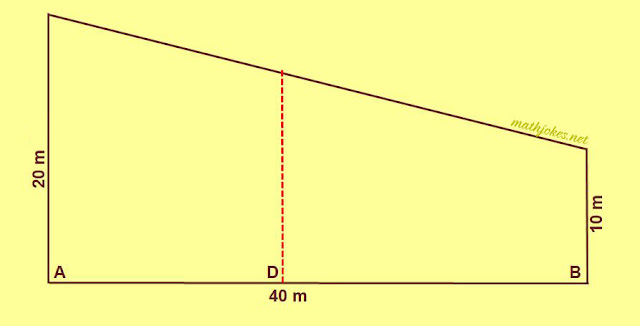# Math Puzzles : Dividing The Board

## Geometrical Puzzles : Dividing The BoardBob had a board measuring 40 feet in length, 10 inches wide at one end, and 20 inches wide at the other, as shown in the illustration. How far from A must the straight cut at D be made in order to divide it into two pieces of equal area?

## 1 comment for "Math Puzzles : Dividing The Board"

1.The Chess Board is contained 64 squares in a took care of set, 8 squares by 8 squares. electronic chess board